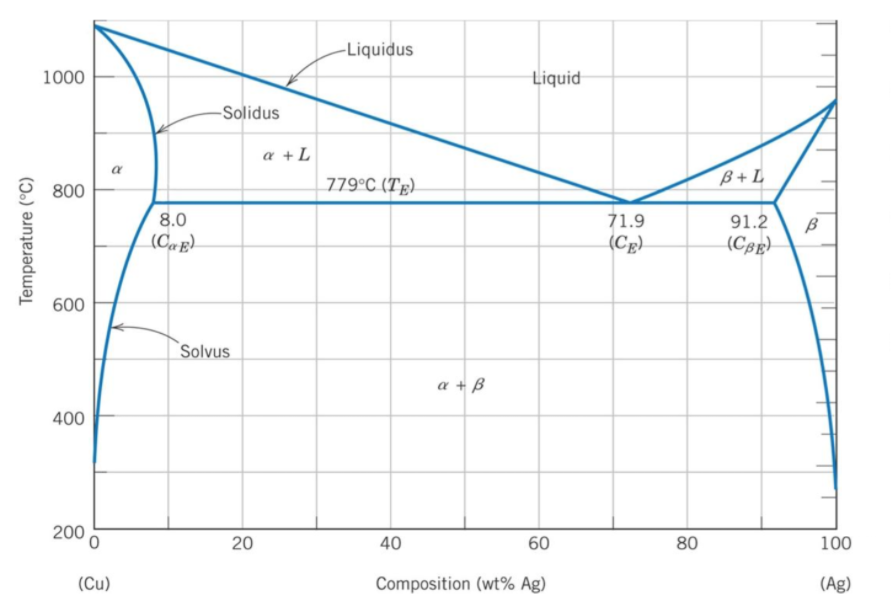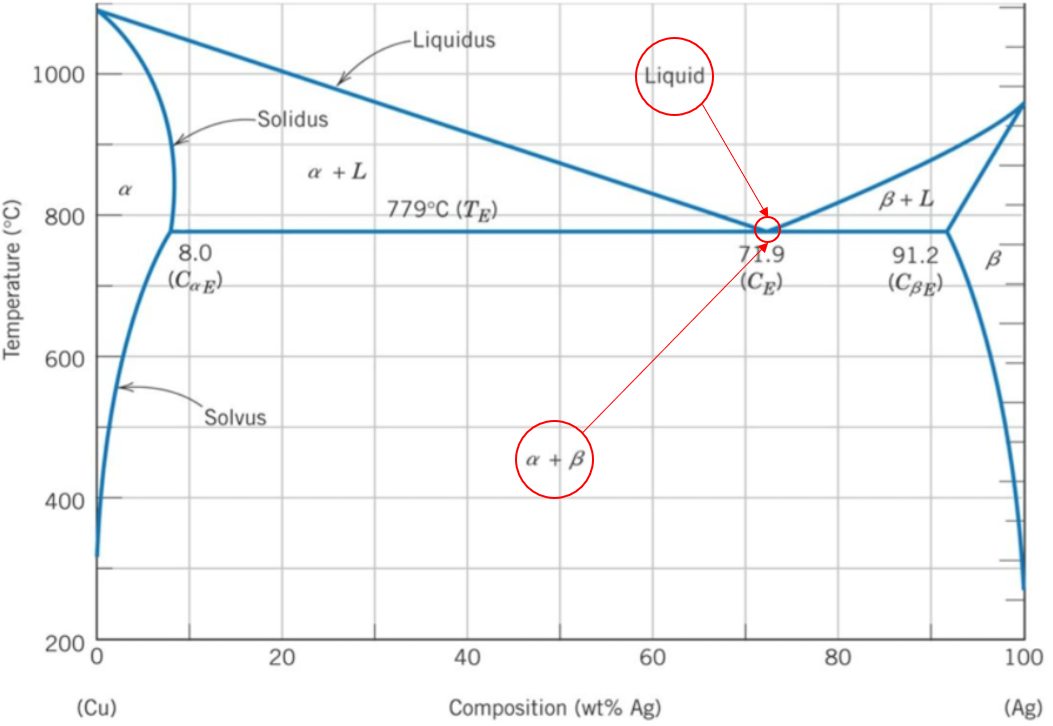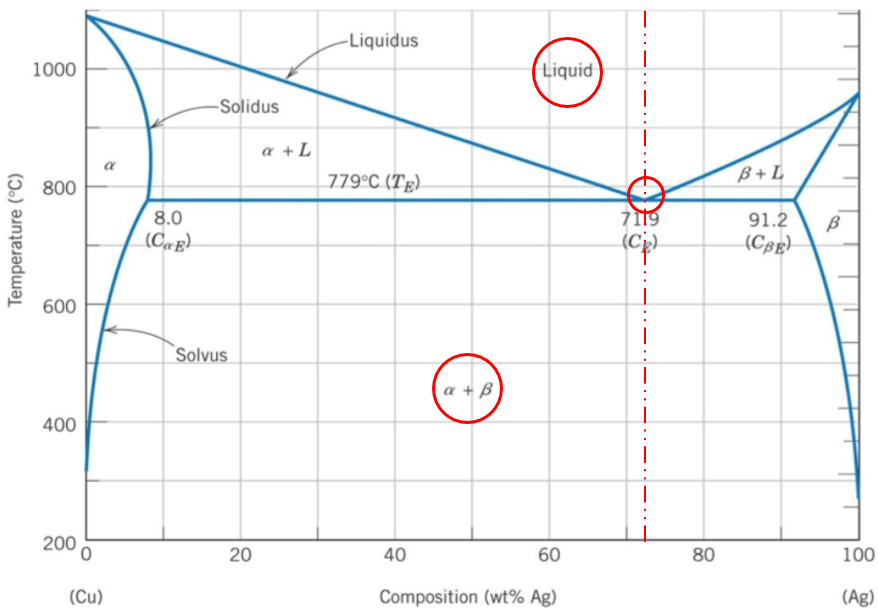## Eutectic Composition

In the phase diagram below, what percentage composition of Ag-Cu will the alloy be in a eutectic composition?Hint
The eutectic composition is the point where an alloy will transform from a liquid phase directly into two solid phases.
Hint 2A binary phase diagram shows the phases (solid, liquid etc) formed by mixing two different elements over a range of temperatures. The eutectic composition is the point where an alloy will transform from a liquid phase directly into two solid phases. In other words, it is the lowest temperature point where the alloy will be completely melted. Based on the Ag-Cu phase diagram, the $$Liquid\rightarrow \alpha + \beta$$ occurs at the 71.9% Ag (100%-71.9% Ag = 28.1% Cu) mark.71.9 wt% Ag